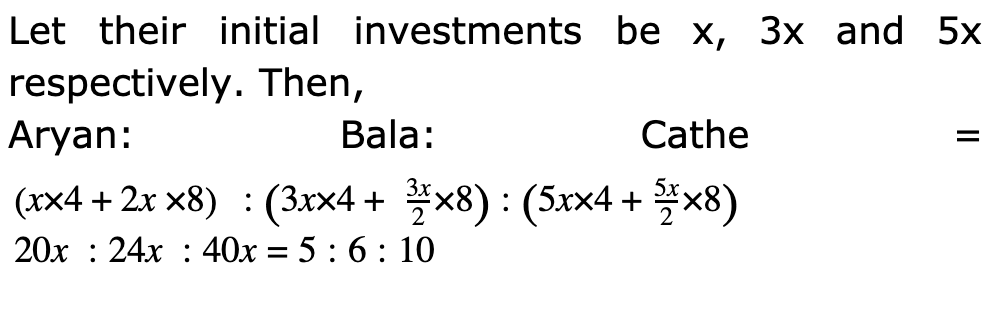CAT | Tech Mahindra Logical Questions

## Tech Mahindra Logical QuestionsJobs By Batch
Jobs By Location
Jobs By Degree
Jobs By Branch
IT Jobs
Internships
Govt. Jobs• ##### Original Questions of IT Companies| All Topics### Quiz Begins Here

Q #1
:

#### Logical Question

Directions: Read the following information to answer the question.

Aryan, Bala, Cathe started a business with their investments in the ratio 1:3 :5. After 4 months, A invested the same amount as before and B as well as C withdrew half of their investments. The ratio of their profits at the end of the year is :
+Q #2
:

#### Logical Question

Directions: Read the following information to answer the question.

Joseph and Kerry are partners in a business. Joseph contributes 1/4 of the capital for 15 months and Kerry received 2/3 of the profit. For how long Kerry's money was used ?
+

Let the total profit be Rs. z. Then,

Kerry's share = Rs. 2z/3,  Joseph’s share = Rs. ( z - 2z/3 ) = Rs. z/3.

J : K = z/3 : 2z/3 = 1:2

Let the total capital be Rs, X and suppose Kerry's money was used for x months. Then.

(1(x) / 4 * 15) / (3x) / 4 * y) = 1/2 <=> y = (15 * 2 / 3) = 10.

Thus, Kerry's money was used for 10 months.

Q #3
:

#### Logical Question

Directions: Read the following information to answer the question.

If the price of gold increases by 50%, find by how much the quantity of ornaments must be reduced, so that the expenditure may remain the same as before?
+

100

150

------

150-------50

100-------? => 331/3%

Q #4
:

#### Logical Question

Directions: Read the following information to answer the question.

Salaries of Jack and Jenny are in the ratio 2: 3. If the salary of each is increased by Rs. 4000, the new ratio becomes 40: 57. What is Jenny�s salary?
+

Let the original salaries of Jack and Jenny’s be Rs. 2x and Rs. 3x respectively.

Then, (2x + 4000)/(3x + 4000) = 40/57

57(2x + 4000) = 40(3x + 4000)

6x = 68,000

3x = 34,000

Jenny's present salary = (3x + 4000) = Rs. (34000 + 4000) = Rs. 38,000.

Q #5
:

#### Logical Question

Directions: Read the following information to answer the question.

What is the fourth proportional of 3 , 5 & 6 ?
+

3/5 = 6 /x . X = 10